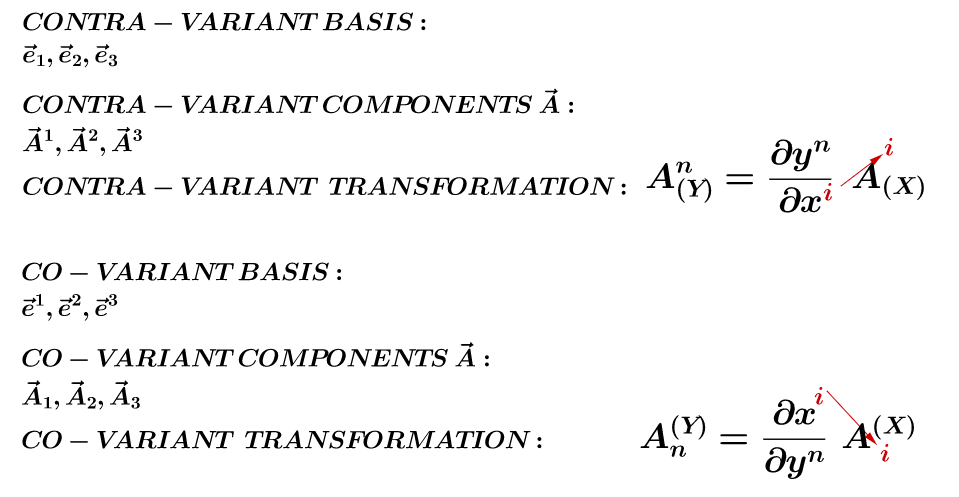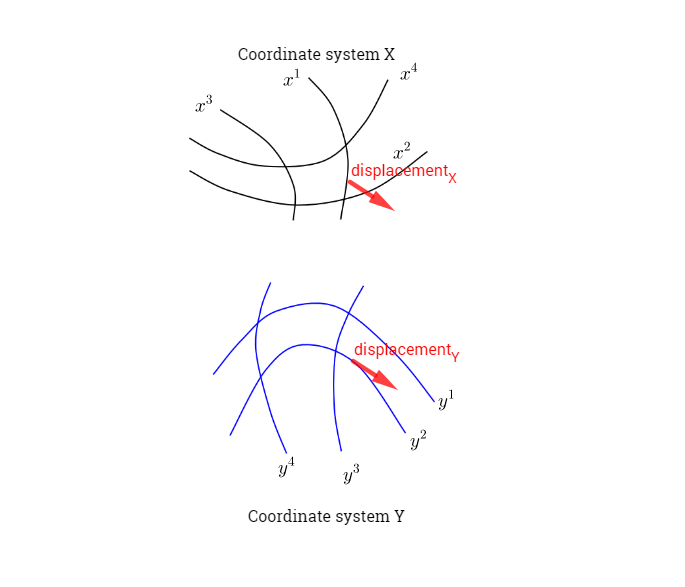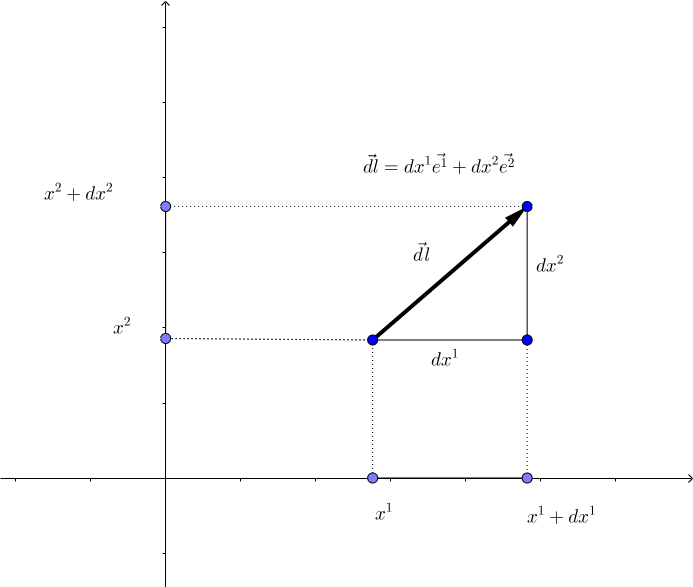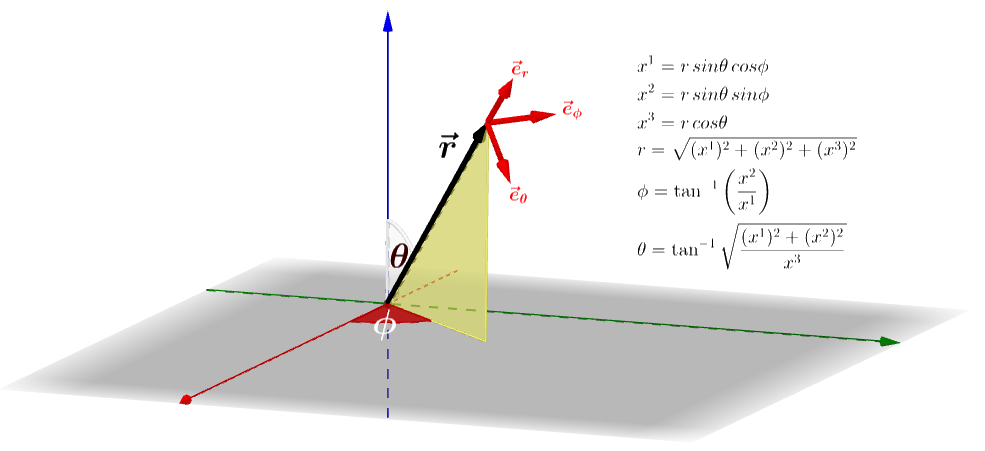#### TENSORS, TANGENT AND ORTHOGONAL VECTORS IN GENERALIZED CURVILINEAR COORDINATES:

##### Abstract:

Vector is a physical quantity and it does not depend on any co-ordinate system. It need to be expanded in some basis for practical calculation and its components do depend on the chosen basis. The expansion in orthonormal basis is mathematically simple. But in many physical situations we have to choose an non-orthogonal basis (or oblique co-ordinate system). But the expansion of a vector in non-orthogonal basis is not convenient to work with. With the notion of contravariant and covariant components of a vector, we make non-orthogonal basis to behave like orthonormal basis.

We introduce $$\vec a = e_1, \; \vec b=e_2,\; \vec c=e_3$$ for contravariant basis and $$\vec a' = e^1, \; \vec b'=e^2,\; \vec c'=e^3$$ for covariant basis. With this notation equation:

$\vec a\cdot \vec a' = \vec b\cdot \vec b'=\vec c\cdot \vec c'=1;\; \vec a\cdot \vec b'=\vec a\cdot \vec c'=0;\;\vec b\cdot \vec a'=\vec b\cdot \vec c'=0;\;\vec c\cdot \vec a'=\vec c\cdot \vec b'=0$

becomes $$I = e_\mu e^\mu\tag {23}$$

and equation

$I = \vec a \vec a' + \vec b \vec b' + \vec c \vec c'$

becomes $$e_i\cdot e^j =\delta_i^j\tag {24}$$

where summation over dummy indices is understood. $$\delta_i^j$$is standard Kronecker delta function. With the introduction of superscript and subscript notation we generalise equation (23) and equation (24) to n-dimensional Euclidean space. The contravariant component of any arbitrary vector $$\vec A$$ is $$A^i$$ with superscript index and covariant component is $$A_i$$ with subscript index are taken to be understood. The dimension of contravariant vector is the inverse of the covariant vector and hence we expect the behaviour of contravariant vector and covariant vector under co-ordinate transformation inverse to each other.KEY point: In a Cartesian system, covariant and contravariant components are the same.

Imagining a differential displacement vector in two different coordinate systems, $$X$$ and $$Y:$$What follows is prdicated on the assumption that we know the equations relating each component ($$m$$) in the $$X$$ coordinate system to the $$Y$$ coordinate frame:

$$Y^n = f(X^m)$$ and $$X^p = g(Y^z).$$

The change in coordinates of the differential displacement vector knowing the transformation equations is given by:

\begin{align} dy^1 &= \frac{\partial y^1}{\partial x^1} dx^1 + \frac{\partial y^1}{\partial x^2} dx^2 + \frac{\partial y^1}{\partial x^3} dx^3 + \cdots + \frac{\partial y^1}{\partial x^n} dx^n\\ dy^2 &= \frac{\partial y^2}{\partial x^1} dx^1 + \frac{\partial y^2}{\partial x^2} dx^2 + \frac{\partial y^2}{\partial x^3} dx^3+\cdots+\frac{\partial y^2}{\partial x^n} dx^n\\ \vdots\\ dy^d &= \frac{\partial y^d}{\partial x^1} dx^1 + \frac{\partial y^d}{\partial x^2} dx^2 + \frac{\partial y^d}{\partial x^3} dx^3+\cdots+\frac{\partial y^d}{\partial x^n} dx^n \end{align}

So any particular component in the new coordinate system would be of the form:

$dy^n = \frac{\partial y^n}{\partial x^\color{blue}{m}} dx^{\color{blue}{m}} \tag{Ref.1}$

with the color coding indicating Einstein’s convention.

Expressed in matrix form:

$\begin{bmatrix} dy^1\\dy^2\\dy^3\\\vdots\\dy^d \end{bmatrix}= {\begin{bmatrix} \frac{\partial y^1}{\partial x^1} & \frac{\partial y^1}{\partial x^2} & \frac{\partial y^1}{\partial x^3} &\cdots& \frac{\partial y^1}{\partial x^n}\\ \frac{\partial y^2}{\partial x^1} & \frac{\partial y^2}{\partial x^2} & \frac{\partial y^3}{\partial x^3} &\cdots& \frac{\partial y^n}{\partial x^n}\\ \vdots&\vdots&\vdots&&\vdots\\ \frac{\partial y^d}{\partial x^1} & \frac{\partial y^d}{\partial x^2} & \frac{\partial y^d}{\partial x^3} &\cdots& \frac{\partial y^d}{\partial x^n}\\ \end{bmatrix}} \large\color{red}{\begin{bmatrix} dx^1\\dx^2\\dx^3\\\vdots\\dx^n \end{bmatrix}}$

We can generalize to a vector $$V$$ (column vector in red), which can be transfored from $$X$$ to $$Y$$ coordinate systems as:

$\bbox[yellow, 5px]{V^n_{(Y)} = \frac{\partial y^{n}}{\partial x^{\color{red}{m}}}\;V^{\color{red}{m}}_{(X)}}$

Notice that in this case $$n = d$$ (the $$d$$ in the matrix above), while $$m$$ is a dummy index, but it is in this case equal to $$n$$. So $$n = d$$ is the dimension of the vector in $$Y$$, or the number of rows of the transformation matrix; and $$m$$ is the number of columns, or the dimension of the vector in the $$X$$ coordinate system.

If we can find the vector component $$n$$ in $$Y$$ of $$V$$ by taking these types of derivatives, we talk about a contravariant vector. The components are expressed as a superscript.

Now let’s take two contravariant vectors: $$A_Y^m$$ ($$m$$-th component of the $$A$$ vector in the $$Y$$ frame): $$\large A_{(Y)}^m= \frac{\partial y^m}{\partial x^r} A_{(X)}^r$$; and the second vector $$\large B_{(Y)}^n= \frac{\partial y^n}{\partial x^s} B_{(X)}^s.$$

If we multiply these two vectors together:

$\large A^m_{(Y)} B^n_{(Y)}$

we have to take the $$d$$ number of components that $$A$$ has and modify each one by the $$d$$ number of components $$B$$ has, expressing it as:

$\large \bbox[10px, border:2px solid red]{T^{mn}_{(Y)} = \large A^m_{(Y)} B^n_{(Y)} =\frac{\partial y^m}{\partial x^r} \; \frac{\partial y^n}{\partial x^s}\; A_{(X)}^r\; B_{(X)}^s= \frac{\partial y^m}{\partial x^\color{blue}{r}} \; \frac{\partial y^n}{\partial x^\color{blue}{s}}\;T^{\color{blue}{rs}}_{(X)}}.$

These are contravariant tensors of the second rank. The $$m,n,r,s$$ superscript are the vector components (elements or entries), while $$(X),(Y)$$ are coordinate systems. So we note that tensors enter when there is a transformation between coordinate systems of more than one vector. This is consistent with the Wikipedia entries both of vectors as multilinear maps:

A downside to the definition of a tensor using the multidimensional array approach is that it is not apparent from the definition that the defined object is indeed basis independent, as is expected from an intrinsically geometric object. Although it is possible to show that transformation laws indeed ensure independence from the basis, sometimes a more intrinsic definition is preferred. One approach is to define a tensor as a multilinear map. In that approach a type $$(p, q)$$ tensor $$T$$ is defined as a map,

$T:\underbrace{V^{*}\times \dots \times V^{*}}_{p{\text{ copies}}}\times \underbrace{V\times \dots \times V}_{q{\text{ copies}}}\rightarrow \mathbf {R}$

where $$V$$ is a (finite-dimensional) vector space and $$V^∗$$ is the corresponding dual space of covectors, which is linear in each of its arguments.

By applying a multilinear map $$T$$ of type $$(p, q)$$ to a basis $$\{e_j\}$$ for $$V$$ and a canonical cobasis $$\{\epsilon^i\}$$ for $$V^∗$$,

$T_{j_{1}\dots j_{q}}^{i_{1}\dots i_{p}}\equiv T(\mathbf{\varepsilon }^{i_{1}},\ldots ,\mathbf {\varepsilon }^{i_{p}},\mathbf{e}_{j_{1}},\ldots ,\mathbf{e}_{j_{q}})$

a $$(p + q)$$-dimensional array of components can be obtained.

However, the most fitting definition is as multidimensional arrays:

Just as a vector in an n-dimensional space is represented by a one-dimensional array of length n with respect to a given basis, any tensor with respect to a basis is represented by a multidimensional array. For example, a linear operator is represented in a basis as a two-dimensional square n × n array. The numbers in the multidimensional array are known as the scalar components of the tensor or simply its components. They are denoted by indices giving their position in the array, as subscripts and superscripts, following the symbolic name of the tensor. For example, the components of an order $$2$$ tensor $$T$$ could be denoted $$T_{ij}$$, where $$i$$ and $$j$$ are indices running from $$1$$ to $$n$$, or also by $$T_i^j$$. Whether an index is displayed as a superscript or subscript depends on the transformation properties of the tensor, described below. The total number of indices required to identify each component uniquely is equal to the dimension of the array, and is called the order, degree or rank of the tensor. However, the term “rank” generally has another meaning in the context of matrices and tensors.

Just as the components of a vector change when we change the basis of the vector space, the components of a tensor also change under such a transformation. Each tensor comes equipped with a transformation law that details how the components of the tensor respond to a change of basis. The components of a vector can respond in two distinct ways to a change of basis (see covariance and contravariance of vectors), where the new basis vectors $$\displaystyle \mathbf {\hat {e}} _{i}$$ are expressed in terms of the old basis vectors $$\displaystyle \mathbf {e} _{j}$$ as,

$\displaystyle \mathbf {\hat {e}}_{i}=\sum_{j=1}^{n}\mathbf {e}_{j}R_{i}^{j}=\mathbf {e} _{j}R_{i}^{j}.$

Here $$R^j_i$$ are the entries of the change of basis matrix, and in the rightmost expression the summation sign was suppressed: this is the Einstein summation convention. The components $$v^i$$ of a column vector $$v$$ transform with the inverse of the matrix $$R$$,

$\displaystyle {\hat {v}}^{i}=(R^{-1})_{j}^{i}v^{j},$

where the hat denotes the components in the new basis. This is called a contravariant transformation law, because the vector transforms by the inverse of the change of basis. In contrast, the components, $$w_i$$, of a covector (or row vector), $$w$$ transform with the matrix $$R$$ itself,

$\displaystyle {\hat {w}}_{i}=w_{j}R_{i}^{j}.$

This is called a covariant transformation law, because the covector transforms by the same matrix as the change of basis matrix. The components of a more general tensor transform by some combination of covariant and contravariant transformations, with one transformation law for each index. If the transformation matrix of an index is the inverse matrix of the basis transformation, then the index is called contravariant and is traditionally denoted with an upper index (superscript). If the transformation matrix of an index is the basis transformation itself, then the index is called covariant and is denoted with a lower index (subscript).

To move on to covariant tensors it is necessary to discuss what a gradient vector is:So if a scalar $$\varphi$$ is a function of $$X^1$$ and $$X^2$$, and we see a differential displacement $$\vec{dl}$$, the change in $$\varphi$$ will be given by:

$\underset{\color{red}{\text{SCALAR}}}{\underbrace{\Huge{d\varphi}}} =\underset{\text{grad. vec.}}{\underbrace{\frac{\partial \varphi}{\partial x^1}}}\,dx^1 + \underset{\text{grad. vec.}}{\underbrace{\frac{\partial \varphi}{\partial x^2}}}\,dx^2\tag 1$

KEY POINT: The gradient vector is in the dual space, taking in a “regular” vector and producing a scalar. In the case of the contravariant vector, a vector in a coordinate frame was transformed into another vector in a different frame.

We also have that

$\vec{dl}= dx^1 \vec{X^1} + dx^2 \vec{X^2}\tag 2$

with $$\vec{X^1}$$ and $$\vec{X^2}$$ representing the unit vectors.

We want a vector that dotted with equation $$(2)$$ results in equation $$(1).$$ Keeping in mind that $$\vec{X^1}$$ and $$\vec{X^2}$$ are unit vectors, the vector we are looking for is the gradient of the scalar $$\varphi$$:

$\vec \nabla \varphi= \frac{\partial \varphi}{\partial x^1}\,\vec{X^1} + \frac{\partial \varphi}{\partial x^2}\,\vec{X^2}\tag 3$

Here’s the dot product:

$d\varphi=\vec{dl}\,\vec{\nabla}\varphi=\color{brown}{ \begin{bmatrix}dx^1 \vec{X^1} & dx^2 \vec{X^2} \end{bmatrix} \begin{bmatrix} \frac{\partial \varphi}{\partial x^1}\,\vec{X^1} \\ \frac{\partial \varphi}{\partial x^2}\,\vec{X^2} \end{bmatrix}}=\frac{\partial \varphi}{\partial x^1}\,dx^1 + \frac{\partial \varphi}{\partial x^2}\,dx^2$

So,

$d\varphi = \vec{dl}\,\vec{\nabla}\varphi$

Generalizing equation $$(3)$$,

$\vec\nabla\varphi=\underset{coord. comp. grad. vec.}{\underbrace{\Large{\frac{\partial\varphi}{\partial x^\color{blue}{m}}}}}\;\vec{X^\color{blue}{m}}$

is the expression of the gradient in the $$X$$ coordinate frame. In the $$Y$$ coordinate frame it would be:

$\vec\nabla\varphi=\Large{\frac{\partial\varphi}{\partial y^\color{blue}{n}}}\;\vec{Y^\color{blue}{n}}$

Applying the chain rule:

$\color{red}{\frac{\partial \varphi}{\partial y^n}}= \frac{\partial \varphi}{\partial x^m} \frac{\partial x^m}{\partial y^n}=\frac{\partial x^m}{\partial y^n}\color{red}{\frac{\partial \varphi}{\partial x^m}}$

This last equation relates the components of the gradient vector in the $$X$$ coordinate frame to the components in the $$Y$$ frame.

Notice that the arrangement of the dummy indices is:

$\frac{\partial \varphi}{\partial y^n}= \frac{\partial x^{\color{red}{m}}}{\partial y^n}\frac{\partial \varphi}{\partial x^{\color{red}{m}}}$

In matrix form:

$\begin{bmatrix} \frac{\partial \varphi}{\partial y^1}\\\frac{\partial \varphi}{\partial y^2}\\\frac{\partial \varphi}{\partial y^3}\\\vdots\\\frac{\partial \varphi}{\partial y^d} \end{bmatrix}= {\begin{bmatrix} \frac{\partial x^1}{\partial y^1} & \frac{\partial x^2}{\partial y^1} & \frac{\partial x^3}{\partial y^1} &\cdots& \frac{\partial x^n}{\partial y^1}\\ \frac{\partial x^1}{\partial y^2} & \frac{\partial x^2}{\partial y^2} & \frac{\partial x^3}{\partial y^2} &\cdots& \frac{\partial x^n}{\partial y^2}\\ \vdots&\vdots&\vdots&&\vdots\\ \frac{\partial x^1}{\partial y^d} & \frac{\partial x^2}{\partial y^d} & \frac{\partial x^3}{\partial y^d} &\cdots& \frac{\partial x^n}{\partial y^d}\\ \end{bmatrix}} \large\color{red}{\begin{bmatrix} \frac{\partial \varphi}{\partial x^1}\\\frac{\partial \varphi}{\partial x^2}\\\frac{\partial \varphi}{\partial x^3}\\\vdots\\\frac{\partial \varphi}{\partial x^n} \end{bmatrix}}$

This arrangement (red column vector - a gradient vector in coordinate system $$X$$) is the form that defines covariant vectors - for example $$W:$$

$\bbox[yellow, 5px]{W^{(Y)}_n = \frac{\partial x^{\color{red}{m}}}{\partial y^n}\, W^{(X)}_{\color{red}{m}}}$

Their components transform from one to another coordinate system like gradient vectors do. The components are subscripts!

Let´s say we have two covariant vectors $$A$$ and $$B$$ with $$d$$ components:

$C_m^{(y)}=\frac{\partial x^r}{\partial y^m} C_r^{(x)}$

$D_n^{(y)}=\frac{\partial x^s}{\partial y^n} D_s^{(x)}$

Multiplying them,

$C_m^{(y)}D_n^{(y)}=\frac{\partial x^r}{\partial y^m}{(y)}\frac{\partial x^s}{\partial y^n}C_r^{(x)}D_s^{(x)}$

$\Large \bbox[10px, border:2px solid red]{T_{mn}^{\small(Y)}= \frac{\partial x^{\color{blue}{r}}}{\partial y^m}\frac{\partial x^{\color{blue}{s}}}{\partial y^n}T_{\color{blue}{rs}}^{(x)}}$

This is a covariant tensor!

There are mixed tensors, such as:

$\Large T^n_m{\small (Y)} =\frac{\partial x^{\color{red}{r}}}{\partial y^m}\frac{\partial y^n}{\partial x^{\color{blue}{s}}}T^{\color{blue}{s}}_{\color{red}{r}}\small (X)$

In a generalized curvilinear coordinate system, the three lines in the diagram can represent the magnitude or position of spherical coordinates:with the two angles involved (in $$3$$ dimensional space) assigned to the other two curvilinar coordinates - say $$u_1$$ represents the magnitude in spherical; $$u_2$$ is for the $$\theta$$ angle; and $$u_3$$ stands for $$\phi.$$